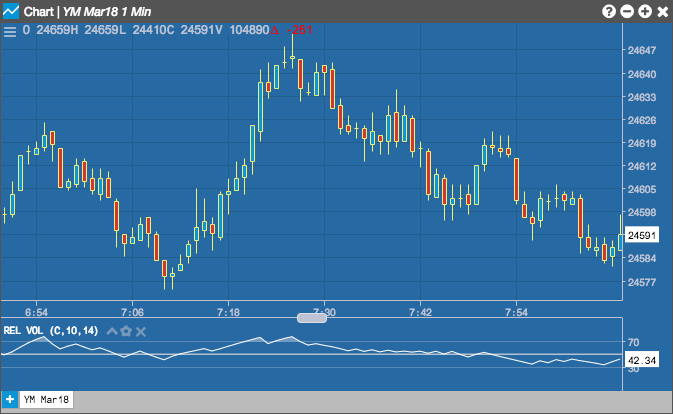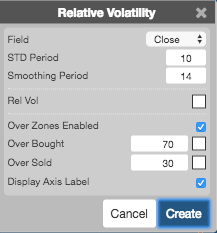Charts

# Relative Volatility

The Relative Volatility indicator measures the standard deviation of price changes within a defined range of lookback periods to determine market direction. The indicator value is normalized as a percentage between 0 and 100.## Configuration Options• Field: Price or combination of prices to use as the base for average calculations. Possible values include:
• Open
• High
• Low
• Close
• HL/2 $$\left ( \frac{High + Low}{2} \right )$$
• HLC/3 $$\left ( \frac{High + Low + Close}{3} \right )$$
• HLCC/4 $$\left ( \frac{High + Low + Close + Close}{4} \right )$$
• OHLC/4 $$\left ( \frac{Open + High + Low + Close}{4} \right )$$
• STD Period: Number of standard deviation periods to use in the calculation.
• Smoothing Period: Number of smoothing periods to use in the calculation.
• Color Selectors: Colors to use for graph elements.
• Over Zones Enabled: Whether to shade the area between the plot and the horizontal overbought and oversold levels.
• Over Bought: Overbought quantity
• Over Sold: Oversold quantity
• Over Bought:
• Over Sold:
• Display Axis Label: Whether to display the most recent value on the Y axis.

## Formula

$RVI = 100 \times \frac{Usum}{(Usum + Dsum)}$

where:

• Usum is the averaged sum of STD for positive days
• Dsum is the averaged sum of STD for negative days